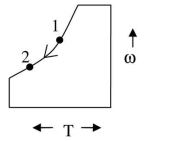## Refrigeration and Air-conditioning Miscellaneous

#### Refrigeration and Air-conditioning

1. Ambient air is at a pressure of 100 kPa, dry bulb temperature of 30° C and 60% relative humidity, The saturation pressure of water at 30° C is 4.24 kPa. The specific humidity of air (in g/kg of dry air) is (correct to two decimal places).

1. Patm = 100 kPa
DBT = t = 30°C → PVS = 4.24 k Pa
φ = 60° = 0.6

 φ PV ⇒ 0.6 = PV PVS 4.24

PV = 2.544 kPa
 w = 0.622 × PV Patm - PV

 w = 0.622 × 2.544 100 - 2.544

w = 16.24 gms/kg of dry air

##### Correct Option: B

Patm = 100 kPa
DBT = t = 30°C → PVS = 4.24 k Pa
φ = 60° = 0.6

 φ PV ⇒ 0.6 = PV PVS 4.24

PV = 2.544 kPa
 w = 0.622 × PV Patm - PV

 w = 0.622 × 2.544 100 - 2.544

w = 16.24 gms/kg of dry air

1. In a mixture of dry air and water vapor at a total pressure of 750 mm of Hg, the partial pressure of water vapor is 20 mm of Hg. The humidity ratio of the air in grams of water vapor per kg of dry air (gw/kgda) is ________

1.  w = 0.622 Pv Pt - Pv

 = 0.622 ×20750 - 20

= 0.017 Kgw/Kgda = 17 gw/Kgda

##### Correct Option: B

 w = 0.622 Pv Pt - Pv

 = 0.622 ×20750 - 20

= 0.017 Kgw/Kgda = 17 gw/Kgda

1. The partial pressure of water vapour in a moist air sample of relative humidity 70% is 1.6 kPa, the total pressure being 101.325 kPa. Moist air may be treated as an ideal gas mixture of water vapour and dry air. The relation between saturation temperature (Ts in K) and saturation pressure (Ps in kPa) for water is given by
 inps= 14.317 - 5304 p0 Ts

where p0 = 101.325 kPa. The dry bulb temperature of the moist air sample (in °C) is_______.

1. φ = 0.7, P0 = 101.325

 φ = Pv ⇒ Psat = Pv Ps - Pw φ

 Psat = 1.6 = 2.28571 kPa 0.7

Corresponding to saturation pressure whatever is the temperature is the DBT
<
 InPs= 14.317 - 5304 P0 Tsat

 ln2.28571= 14.317 - 5304 - 3.7916 = 14.317 - 5304 101.325 Tsat Tsat

 Tsat = 5304 = 292.898 K = 19.89°C 18.1086

##### Correct Option: C

φ = 0.7, P0 = 101.325

 φ = Pv ⇒ Psat = Pv Ps - Pw φ

 Psat = 1.6 = 2.28571 kPa 0.7

Corresponding to saturation pressure whatever is the temperature is the DBT
<
 InPs= 14.317 - 5304 P0 Tsat

 ln2.28571= 14.317 - 5304 - 3.7916 = 14.317 - 5304 101.325 Tsat Tsat

 Tsat = 5304 = 292.898 K = 19.89°C 18.1086

1. Air in a room is at 35°C and 60% relative humidity (RH). The pressure in the room is 0.1 MPa. The saturation pressure of water at 35°C is 5.63 kPa. The humidity ratio of the air (in gram/kg of dry air) is_________.

1.  φ = Pw = 0.6 = Pw Ps 5.63

∴ Pw = 3.378 kPa
Humidity Ratio,
 w = 0.622 Pw = 0.622 × 3.378 Ps - Pw 100 - 3.378

= 0.021745 kg/kg of dry air
or 21.745 g/kg of dry air

##### Correct Option: A

 φ = Pw = 0.6 = Pw Ps 5.63

∴ Pw = 3.378 kPa
Humidity Ratio,
 w = 0.622 Pw = 0.622 × 3.378 Ps - Pw 100 - 3.378

= 0.021745 kg/kg of dry air
or 21.745 g/kg of dry air

1. Moist air at 35°C and 100% relative humidity is entering a psychrometric device and leaving at 25°C and 100% relative humidity. The name of the device is

1.Since water content in moist air is reducing. The device is dehumidifer.

##### Correct Option: BSince water content in moist air is reducing. The device is dehumidifer.